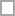# Chemical Calculation Quizzes & Trivia

Chemical Calculations Notes 1 answers to examples. Note: 1. If your answer for number of atoms is (for example) 3.00 x 1023, your answer has to be entered as 3.00 x 10^23 ie. 3.00(space)x(space)10^23 ^ represents...

Questions: 10  |  Attempts: 59   |  Last updated: Jan 3, 2013

Chemical Calculations Notes 2 answers to examples. Note: 1. If your answer for number of atoms is (for example) 3.00 x 1023, your answer has to be entered as 3.00 x 10^23 ie. 3.00(space)x(space)10^23 ^...

Questions: 4  |  Attempts: 42   |  Last updated: Jan 3, 2013

Chemical Calculations Notes 4 answers to examples. Note: 1. If your answer for number of atoms is (for example) 3.00 x 1023, your answer has to be entered as 3.00 x 10^23 ie. 3.00(space)x(space)10^23 ^ represents...

Questions: 7  |  Attempts: 20   |  Last updated: Jan 28, 2013
• Sample Question
Example 16 If 500cm3 of hydrogen and 300cm3 of oxygen were exploded in a bomb calorimeter, and the contents were allowed to cool to room temperature, what is the identity and volume (equivalent at 1atm) of gas remaining in the calorimeter?Chemical Calculations Notes 3 answers to examples. Note: 1. If your answer for number of atoms is (for example) 3.00 x 1023, your answer has to be entered as 3.00 x 10^23 ie....

Questions: 8  |  Attempts: 19   |  Last updated: Jan 28, 2013
• Sample Question
Example 14 Sulfuric acid reacts with zinc carbonate to produce carbon dioxide, water and zinc sulfate. If 100 molecules of sulfuric acid reacted with an excess of zinc carbonate, find the number of carbon dioxide and water molecules formed from the reaction.Chemical Calculations Notes 5 answers to examples. Note: 1. If your answer for number of atoms is (for example) 3.00 x 1023, your answer has to be entered as 3.00 x 10^23 ie....

Questions: 10  |  Attempts: 9   |  Last updated: Jan 28, 2013Related

## Chemical Calculation Questions and Answers

• Example 16 If 500cm3 of hydrogen and 300cm3 of oxygen were exploded in a bomb calorimeter, and the contents were allowed to cool to room temperature, what is the identity and volume (equivalent at 1atm) of gas remaining in the calorimeter?Chemical calculation question from

• Example 17 A student wanted to prepare 3.00g of copper(II) sulfate pentahydrate crystals from reacting copper(II) carbonate with dilute sulfuric acid. If he uses 2.00g of copper(II) carbonate and a solution containing 1.00g of sulfuric acid, would he obtain the desired amount of copper(II) sulfate crystals? Hint: Note that the crystals contain water of crystalllization! Mr of water of crystallization should be added to the Mr of the hydrated crystals.Chemical calculation question from

• Example 14 Sulfuric acid reacts with zinc carbonate to produce carbon dioxide, water and zinc sulfate. If 100 molecules of sulfuric acid reacted with an excess of zinc carbonate, find the number of carbon dioxide and water molecules formed from the reaction.Chemical calculation question from

• Example 14A – Molar Calculations Sulfuric acid reacts with zinc carbonate to produce carbon dioxide, water and zinc sulfate. If 1.25g of zinc carbonate reacted with excess sulfuric acid, find the number of moles of carbon dioxide and zinc sulfate formed from the reaction.Chemical calculation question from